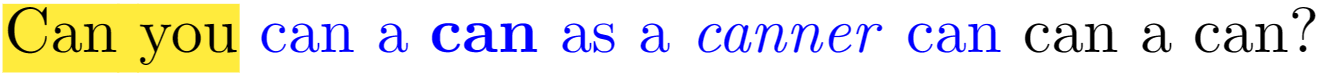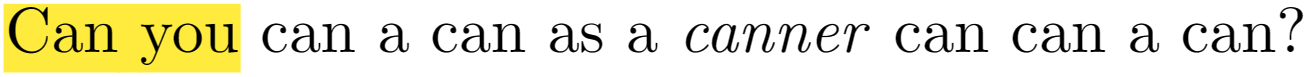# Remove a specific latex command from the text AND closing bracket behind it

How to remove a specific latex command from the text AND closing bracket behind it, but to keep the text inside the brackets/

senseless example:

We \edit{Introduce a} model for analyzing \emph{data} from various
experimental designs, \edit{such as paired or \url{http://www/}
longitudinal; as was done 1984 by NN \cite{mycitation} and by NNN
\cite{mycitation2}}.


Output:

We Introduce a model for analyzing \emph{data} from various
experimental designs, such as paired or \url{http://www/}
longitudinal; as was done 1984 by NN \cite{mycitation} and by NNN
\cite{mycitation2}.


PS. I am introducing a lot of small edits into my tex file. I want those edits to be highlighted, so my collaborator can see them. But afterwards I would like to remove all highlights and to send the text to a reviewer.

The question was originally asked at https://unix.stackexchange.com/questions/373662/awk-sed-remove-a-specific-latex-command-from-the-text-and-closing-bracket-behind. But example there was too soft

• @den2042: do you want to disable the highlighting (see TH's answer) or do you want to change the source, i.e. remove \edit{ and the closing} from it?
– Mike
Jun 27, 2017 at 20:55
• @Teepeemm, there's a second \edit{such as ... \cite{mycitation2}}.
– TH.
Jun 27, 2017 at 21:02
• @Mike I would like to change the source, i.e. remove \edit{ and the closing} from it. The reason for that is there maybe many more consecutive overlapping edits. I would like to send the clean text to each collaborator Jun 27, 2017 at 21:41
• @den2042: that's a job for regular expressions, which I don't know well enough for this, sorry.
– Mike
Jun 27, 2017 at 21:58
• If you would have similar question, please check ilkkachu answer at unix.stackexchange.com/questions/373772/… Jun 27, 2017 at 22:57

Since it sounds like you want to modify your source code, I've written a new nonanswer.

This is not something that you want to be doing from within TeX. You might be tempted to try to write a regular expression for it, but you're not going to succeed using real regular expressions.

A better solution might be to instruct your editor to do what you want. For example, in Vim, with the cursor over the \ in \edit{...}, entering 5xma%xax deletes the first 5 characters (the \edit), marks the current location (the {), moves to the matching }, deletes it, moves back to the marked location and deletes it. Combined with /\\edit{ as part of a VIM macro makes deleting them pretty easy.

Credit to Justin Smith's answer for the ma%xax. (Or as the edit notes, %xx has the same effect as ma%xax.)

This answer assumed you wanted to simply make \edit not do anything which it's now clear is not what you want.

You want to (re)define \edit to just output its argument without change.

\renewcommand\edit{#1}


Here's an example.

\documentclass{article}
\usepackage{xcolor}
\usepackage{url}

\newcommand\edit{\textcolor{blue}{#1}}

%\renewcommand\edit{#1}

\begin{document}
We \edit{Introduce a} model for analyzing \emph{data} from various
experimental designs, \edit{such as paired or \url{http://www/}
longitudinal; as was done 1984 by NN \cite{mycitation} and by NNN
\cite{mycitation2}}.

We Introduce a model for analyzing \emph{data} from various
experimental designs, such as paired or \url{http://www/}
longitudinal; as was done 1984 by NN \cite{mycitation} and by NNN
\cite{mycitation2}.
\end{document}If I uncomment the \renewcommand line, I get the following.In this case, the two paragraphs are identical.

Here is a solution based on Python, not concise enough but performs well with nested commands.

def command_remove(tex_in, keywords):
# Romove command with curly bracket
# keywords: "hl textbf" mean removing \hl{} and \textbf{}
pattern = '\\\\(' + keywords.replace(' ', '|') + '){'
commands = re.finditer(pattern, tex_in)
idxs_to_del = [] # The index of }
for command in commands:
stack = 0
current_loc = command.span()
while not (tex_in[current_loc] == '}' and stack == 0):
if tex_in[current_loc] == '}':
stack = stack - 1
if tex_in[current_loc] == '{':
stack = stack + 1
current_loc = current_loc + 1
idxs_to_del.append(current_loc)

idxs_to_del = sorted(idxs_to_del, reverse=True) # sort
tex_list = list(tex_in)
for idx in idxs_to_del:
tex_list.pop(idx) # remove }

tex_out = ''.join(tex_list)
tex_out = re.sub(pattern, '', tex_out) # remove \xxx{
return tex_out


It locates the target command by regular expression and then locates the position of the closing bracket with a stack. For tex_out = command_remove(tex_in, "revise textbf") with tex_in:

\hl{Can you} \revise{can a \textbf{can} as a \emph{canner} can} can a can?we would get tex_out:

\hl{Can you} can a can as a \emph{canner} can can a can?More details, i.e., command line running, are in Latex_command_remove.

Though an old post, it might still be relevant and interesting.

Here's an approach using package changes from ctan.

First, let's have a look at the original snippet, replacing the \edit{} statements with code from changes, using the [draft] option. I included a \listofchanges at the end, which can be further adjusted via options.\documentclass[10pt]{article}
\usepackage{hyperref}

% ~~~ this one is newly introduced here ~~~~~
\usepackage[draft]{changes}% <<< draft

% ~~~ to define ID's and assign colors ~~~~
\definechangesauthor[color=orange]{DEN}\definechangesauthor[color=red]{ME}

\begin{document}
% ~~~ replacing \edit{ } from original ~~~~
We
model for analyzing \emph{data} from various experimental designs, such as paired or \url{http://www/} longitudinal; as was done 1984 by NN \cite{mycitation}
\deleted[id=ME,comment={just to show this feature}]{and by NNN\cite{mycitation2}}
.

\vspace{2\baselineskip}
\listofchanges

\end{document}


~~

Finally let's have a look at what happens to the same code, when switching to the [final] option for the package: It keeps all changes-code, but reworks the output pdf as needed.\documentclass[10pt]{article}
\usepackage{hyperref}

% ~~~ this one is newly introduced here ~~~~~
\usepackage[final]{changes}% <<< switching to final

% ~~~ to define ID's and assign colors ~~~~
\definechangesauthor[color=orange]{DEN}\definechangesauthor[color=red]{ME}

\begin{document}
% ~~~ replacing \edit{ } from original ~~~~
We

To remove all changes-markups, apply script pyMergeChanges.py`, as described in the manual.